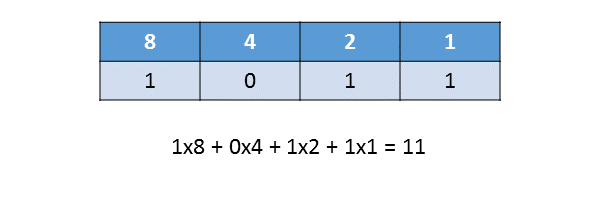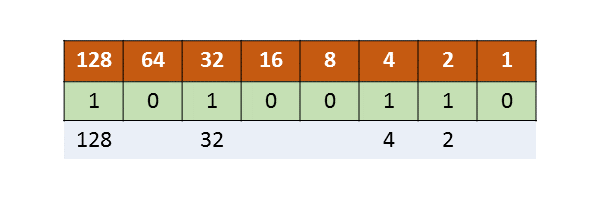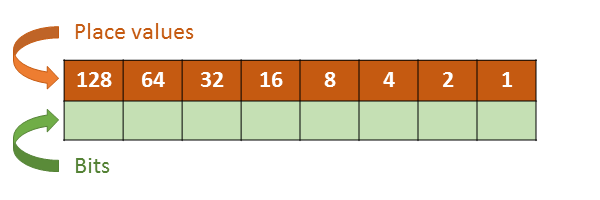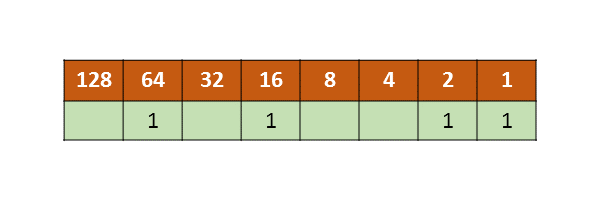# Binary numbers

Martin McBride, 2017-02-21
Tags binary denary place values
Categories data representation numbers

Computers use binary to represent numbers. Binary is similar to our normal denary (base 10) number system, but it uses base 2. This means that place values work slightly differently.

## Denary (base 10) place values

Denary place values are so familiar that you almost don't realise that you are using them. Consider the number 2107:This number is made up of 2 thousands, 1 hundred, no tens and 7 units, which add up to (not surprisingly) 2107.

But to recap with base 10:

• It uses digits 0 to 9
• Each place value is 10 times bigger than the previous (place values are powers of 10)

## Binary (base 2) place values

Binary uses base 2, which means:

• It uses digits 0 and 1
• Each place value is twice as big as the previous (place values are powers of 2)

Here is the binary number 1101:As you can see, the first place represents units, then twos, then fours, then eights, etc. To calculate the value of the number, we add 1 eight, 1 four, no twos and 1 unit, which gives 13.

1101 in binary is equal to 13 in denary.

## Counting in binary

This table show how to count from 0 to 15 in binary. Notice that pattern of 1s and 0s in each column:## Converting binary to denary

To convert a binary number to base 10, draw a simple table like this:The top row shows the place values - starting at 1 (on the right) each place value is 2 times the previous (2, 4, 8, 16 etc). The bottom row holds the binary digits (0 or 1). The table shows the binary number 10100110.

To convert the binary number to denary, we just need to write down the place value of each binary 1 digit, and add them all up. 128 + 32 +4 + 2 equals 166. That is the denary equivalent of binary 10100110.## Converting denary to binary

As an example, we will convert 83 base 10 to a binary number. You can convert denary to binary using the same table, initially empty:The technique is simple. Keep subtracting the biggest power of two that you can, until there is nothing left.

• Starting with 83, the biggest power of 2 we can subtract is 64. Place a one in the "64" column and subtract it.
• This leaves us with 29. The biggest power of 2 we can subtract is 16. Place a one in the "16" column and subtract it.
• This leaves us with 3. The biggest power of 2 we can subtract is 2. Place a one in the "2" column and subtract it.
• This leaves us with 1. The biggest power of 2 we can subtract is 1. Place a one in the "1" column and subtract it.
• Now we are left with zero so we are done.Our final table has ones in the positions shown. The remaining positions can be filled with zeros, giving a final binary value of 01010011.